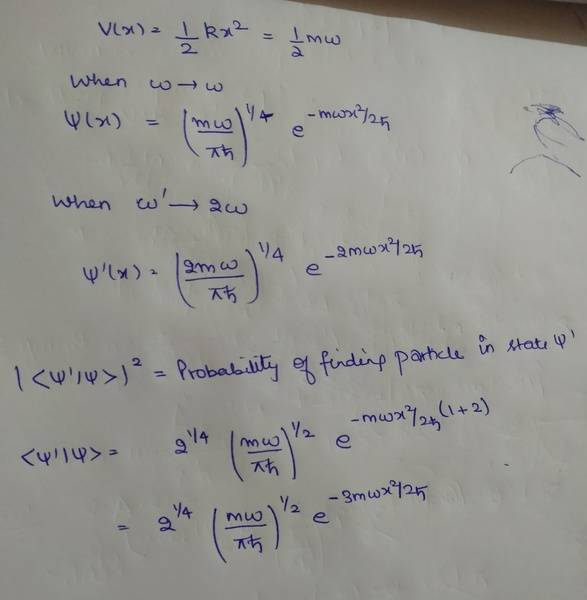# A particle of mass 'm' is initially in a ground state of 1- D Harmonic oscillator potential V(x)...

Sushmita

## Homework Statement

[/B]
A particle of mass 'm' is initially in a ground state of 1- D Harmonic oscillator potential V(x) = (1/2) kx2 . If the spring constant of the oscillator is suddenly doubled, then the probability of finding the particle in ground state of new potential will be?
(A) 21/4/(1+ 21/2)
(B) 25/4/(1+21/2)
(C) 2/(1+21/2)
(D) 23/2/(1+21/2)

## Homework Equations

I calculated state with the wave function of one dimentional harmonic oscillator given by
Ψ = (k/πħ)¼ exp (-kx2/2ħ)

When k was doubled, new wave function becomes

Ψ'= (2k/πħ)¼ exp (-2kx2/2ħ)

## The Attempt at a Solution

I tried solving the question by calculating the probability by finding the inner product of the two but I cannot solve it#### Attachments

Last edited by a moderator:

## Answers and Replies

Staff Emeritus
Homework Helper
Your expression for the ground-state wave function has errors as the argument of the exponential isn't unitless.

Your approach is fine, but we can't really help you if you don't show your calculations.

Sushmita
Your expression for the ground-state wave function has errors as the argument of the exponential isn't unitless.

Your approach is fine, but we can't really help you if you don't show your calculations.

I am attaching my solution in the attachment below.Staff Emeritus